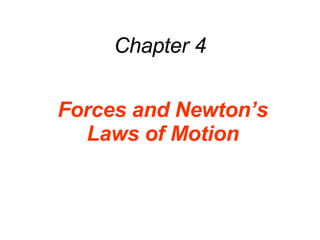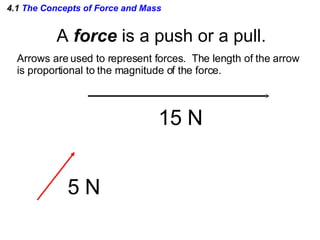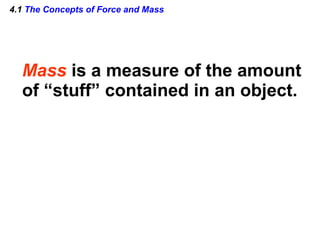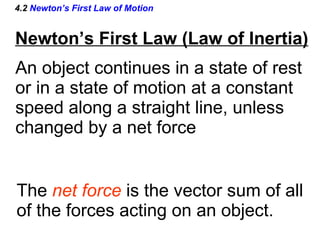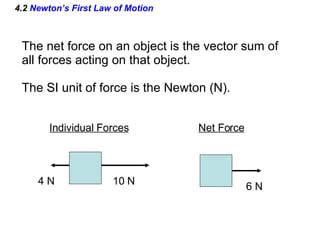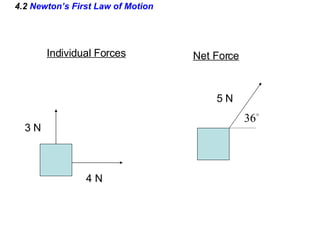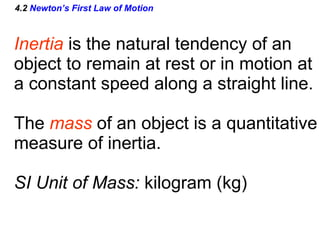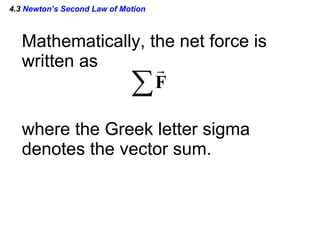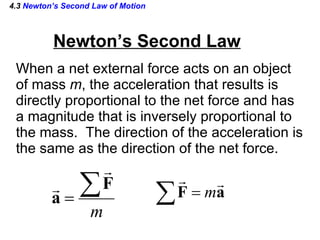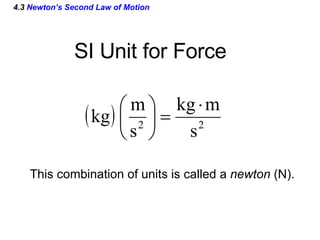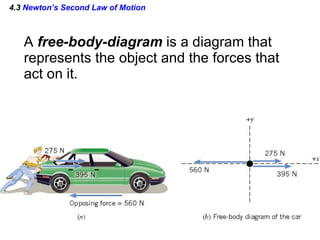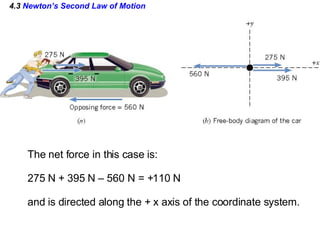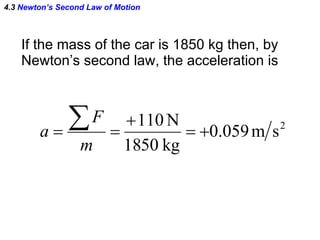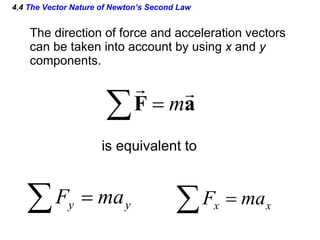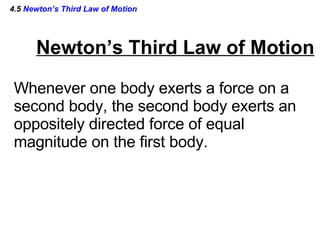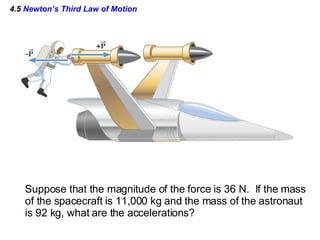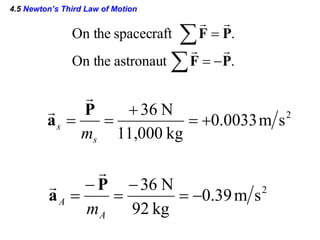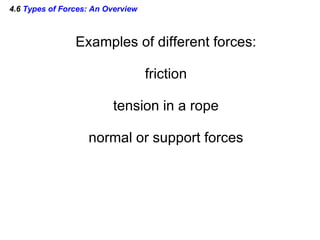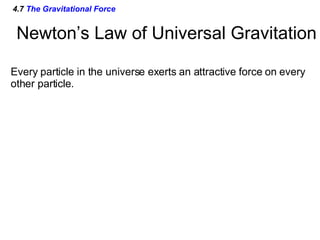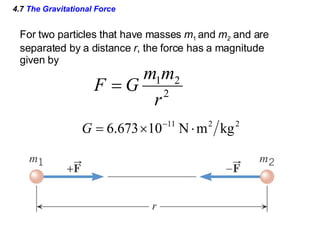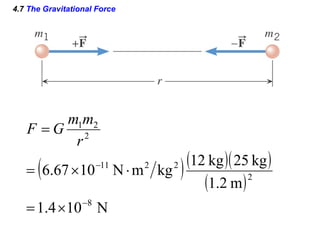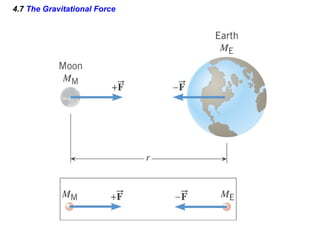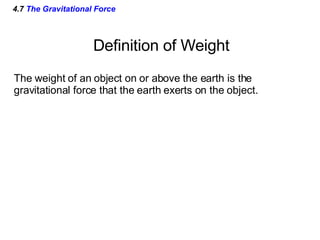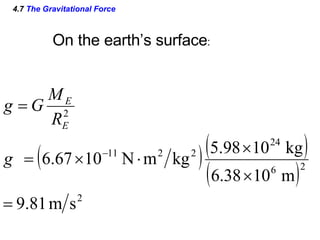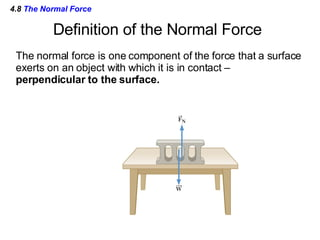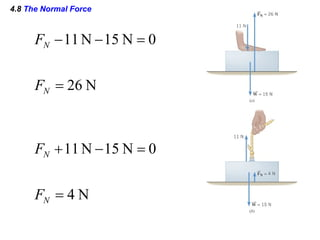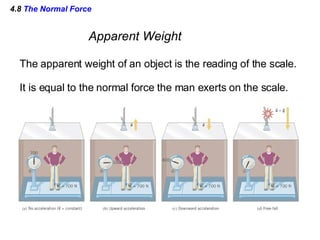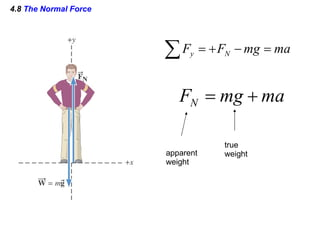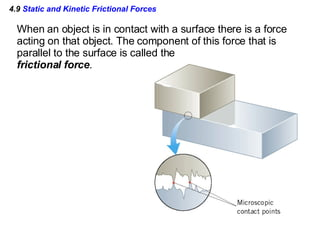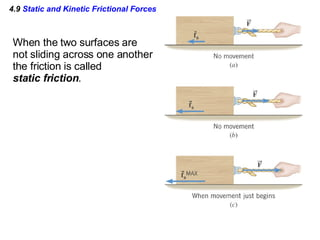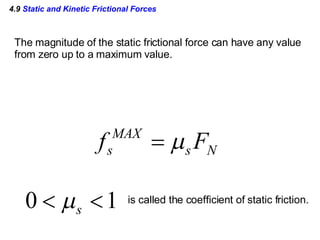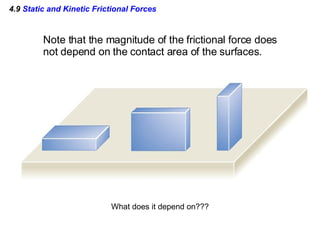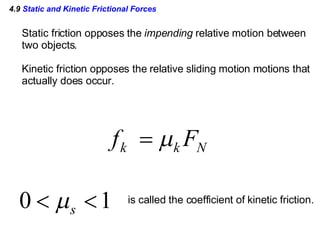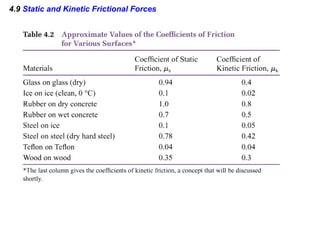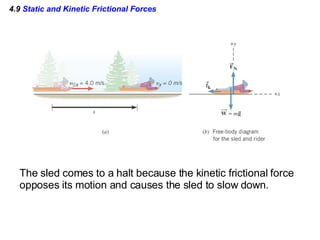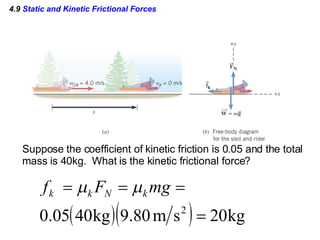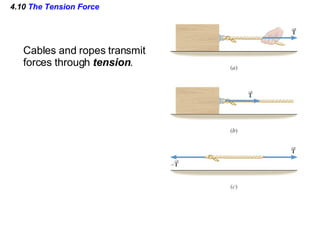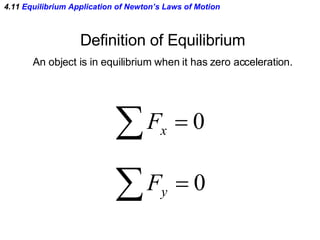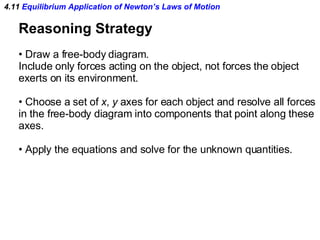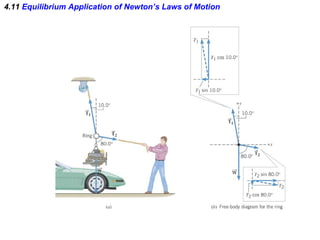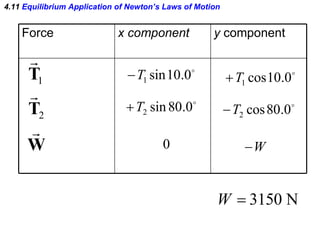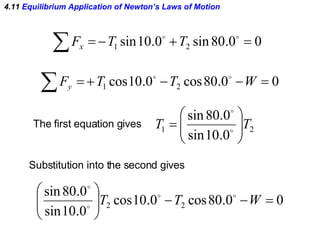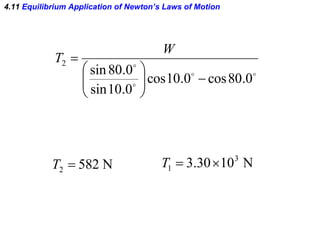1 of 43

### Chapter 4 Powerpoint

1. Chapter 4 Forces and Newton’s Laws of Motion
2. 4.1 The Concepts of Force and Mass A force is a push or a pull. Arrows are used to represent forces. The length of the arrow is proportional to the magnitude of the force. 15 N 5 N
3. 4.1 The Concepts of Force and Mass Mass is a measure of the amount of “stuff” contained in an object.
4. 4.2 Newton’s First Law of Motion An object continues in a state of rest or in a state of motion at a constant speed along a straight line, unless changed by a net force The net force is the vector sum of all of the forces acting on an object. Newton’s First Law (Law of Inertia)
5. 4.2 Newton’s First Law of Motion The net force on an object is the vector sum of all forces acting on that object. The SI unit of force is the Newton (N). Individual Forces Net Force 10 N 4 N 6 N
6. 4.2 Newton’s First Law of Motion Individual Forces Net Force 3 N 4 N 5 N
7. 4.2 Newton’s First Law of Motion Inertia is the natural tendency of an object to remain at rest or in motion at a constant speed along a straight line. The mass of an object is a quantitative measure of inertia. SI Unit of Mass: kilogram (kg)
8. 4.3 Newton’s Second Law of Motion Mathematically, the net force is written as where the Greek letter sigma denotes the vector sum.
9. 4.3 Newton’s Second Law of Motion Newton’s Second Law When a net external force acts on an object of mass m , the acceleration that results is directly proportional to the net force and has a magnitude that is inversely proportional to the mass. The direction of the acceleration is the same as the direction of the net force.
10. 4.3 Newton’s Second Law of Motion SI Unit for Force This combination of units is called a newton (N).
11. 4.3 Newton’s Second Law of Motion A free-body-diagram is a diagram that represents the object and the forces that act on it.
12. 4.3 Newton’s Second Law of Motion The net force in this case is: 275 N + 395 N – 560 N = +110 N and is directed along the + x axis of the coordinate system.
13. 4.3 Newton’s Second Law of Motion If the mass of the car is 1850 kg then, by Newton’s second law, the acceleration is
14. 4.4 The Vector Nature of Newton’s Second Law The direction of force and acceleration vectors can be taken into account by using x and y components. is equivalent to
15. 4.5 Newton’s Third Law of Motion Newton’s Third Law of Motion Whenever one body exerts a force on a second body, the second body exerts an oppositely directed force of equal magnitude on the first body.
16. 4.5 Newton’s Third Law of Motion Suppose that the magnitude of the force is 36 N. If the mass of the spacecraft is 11,000 kg and the mass of the astronaut is 92 kg, what are the accelerations?
17. 4.5 Newton’s Third Law of Motion
18. 4.6 Types of Forces: An Overview Examples of different forces: friction tension in a rope normal or support forces
19. 4.7 The Gravitational Force Newton’s Law of Universal Gravitation Every particle in the universe exerts an attractive force on every other particle.
20. 4.7 The Gravitational Force For two particles that have masses m 1 and m 2 and are separated by a distance r , the force has a magnitude given by
21. 4.7 The Gravitational Force
22. 4.7 The Gravitational Force
23. 4.7 The Gravitational Force Definition of Weight The weight of an object on or above the earth is the gravitational force that the earth exerts on the object.
24. 4.7 The Gravitational Force On the earth’s surface :
25. 4.8 The Normal Force Definition of the Normal Force The normal force is one component of the force that a surface exerts on an object with which it is in contact – perpendicular to the surface.
26. 4.8 The Normal Force
27. 4.8 The Normal Force Apparent Weight The apparent weight of an object is the reading of the scale. It is equal to the normal force the man exerts on the scale.
28. 4.8 The Normal Force apparent weight true weight
29. 4.9 Static and Kinetic Frictional Forces When an object is in contact with a surface there is a force acting on that object. The component of this force that is parallel to the surface is called the frictional force .
30. 4.9 Static and Kinetic Frictional Forces When the two surfaces are not sliding across one another the friction is called static friction .
31. 4.9 Static and Kinetic Frictional Forces The magnitude of the static frictional force can have any value from zero up to a maximum value. is called the coefficient of static friction.
32. 4.9 Static and Kinetic Frictional Forces Note that the magnitude of the frictional force does not depend on the contact area of the surfaces. What does it depend on???
33. 4.9 Static and Kinetic Frictional Forces Static friction opposes the impending relative motion between two objects. Kinetic friction opposes the relative sliding motion motions that actually does occur. is called the coefficient of kinetic friction.
34. 4.9 Static and Kinetic Frictional Forces
35. 4.9 Static and Kinetic Frictional Forces The sled comes to a halt because the kinetic frictional force opposes its motion and causes the sled to slow down.
36. 4.9 Static and Kinetic Frictional Forces Suppose the coefficient of kinetic friction is 0.05 and the total mass is 40kg. What is the kinetic frictional force?
37. 4.10 The Tension Force Cables and ropes transmit forces through tension .
38. 4.11 Equilibrium Application of Newton’s Laws of Motion Definition of Equilibrium An object is in equilibrium when it has zero acceleration.
39. 4.11 Equilibrium Application of Newton’s Laws of Motion
40. 4.11 Equilibrium Application of Newton’s Laws of Motion Force x component y component
41. 4.11 Equilibrium Application of Newton’s Laws of Motion The first equation gives Substitution into the second gives
42. 4.11 Equilibrium Application of Newton’s Laws of Motion